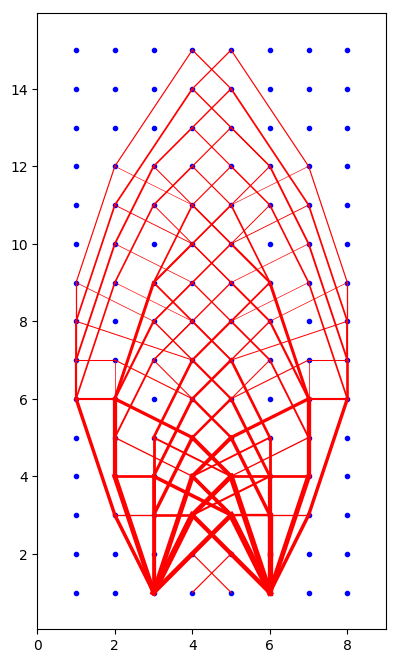In :
### MAKING A BRIDGE

Nx = 15
Ny = 7

# FIXED NODES
fixed = [ sub2ind((Nx,Ny),1,1), sub2ind((Nx,Ny),Nx,1)]

# uniform load
loaded = [ (sub2ind((Nx,Ny),j,1), 0,1) for j = 1:Nx ]

# point load
loaded = [ (sub2ind((Nx,Ny),Int((Nx+1)/2),1), 0,Nx) ];

In :
### MAKING A TOWER

Nx = 8
Ny = 15

# FIXED NODES
fixed = [ sub2ind((Nx,Ny),i,1) for i = 3:6 ]

# crunching
#loaded = [ (sub2ind((Nx,Ny),i,Ny), 0,1) for i = 4:5 ]

# uniform lateral load
loaded1 = [ (sub2ind((Nx,Ny),4,j), 1,0) for j = 1:Ny ];
loaded2 = [ (sub2ind((Nx,Ny),5,j), 1,0) for j = 1:Ny ];
loaded = [loaded1; loaded2]

Out:
30-element Array{Tuple{Int64,Int64,Int64},1}:
(4, 1, 0)
(12, 1, 0)
(20, 1, 0)
(28, 1, 0)
(36, 1, 0)
(44, 1, 0)
(52, 1, 0)
(60, 1, 0)
(68, 1, 0)
(76, 1, 0)
(84, 1, 0)
(92, 1, 0)
(100, 1, 0)
⋮
(29, 1, 0)
(37, 1, 0)
(45, 1, 0)
(53, 1, 0)
(61, 1, 0)
(69, 1, 0)
(77, 1, 0)
(85, 1, 0)
(93, 1, 0)
(101, 1, 0)
(109, 1, 0)
(117, 1, 0)
In :
N = Nx*Ny  # number of nodes

# NODES: columns are x and y components respectively
nodes = [ kron(ones(Ny),collect(1:Nx)) kron(collect(1:Ny),ones(Nx)) ]

M = Int(N*(N-1)/2)  # number of edges

# EDGES: columns are the indices of the nodes at either end
edges = Array{Int}(zeros(M,2))

k = 0
for i = 1:N
for j = 1:i-1
k = k+1
edges[k,:] = [i j]
end
end

ℓ = zeros(M)
nx = zeros(N,M)
ny = zeros(N,M)
for j = 1:M
i1 = edges[j,1]
i2 = edges[j,2]
ℓ[j] = norm( [nodes[i1,1]-nodes[i2,1], nodes[i1,2]-nodes[i2,2]] )
nx[i1,j] = (nodes[i1,1]-nodes[i2,1])/ℓ[j]
nx[i2,j] = (nodes[i2,1]-nodes[i1,1])/ℓ[j]
ny[i1,j] = (nodes[i1,2]-nodes[i2,2])/ℓ[j]
ny[i2,j] = (nodes[i2,2]-nodes[i1,2])/ℓ[j]
end

fx = zeros(N)
fy = zeros(N)
for L in loaded
ind = L
fx[ind] = L
fy[ind] = L
end

using JuMP, Gurobi
m = Model(solver=GurobiSolver(OutputFlag=0))

@variable(m, x[1:M] >= 0)   # area of edge from i to j
@variable(m, u[1:M] )       # force in edge from i to j

for i = 1:N
if i in fixed
continue
else
@constraint(m, sum(u[j]*nx[i,j] for j=1:M) + fx[i] == 0 )
@constraint(m, sum(u[j]*ny[i,j] for j=1:M) + fy[i] == 0 )
end
end

@constraint(m, -x .<= u)
@constraint(m,  u .<= x)

@objective(m, Min, sum(ℓ[j]*x[j] for j=1:M))

solve(m)
xopt = getvalue(x);
uopt = getvalue(u);

using PyPlot

figure(figsize=((Nx+1)/2,(Ny+1)/2))

plot( nodes[:,1], nodes[:,2], "b." )
for j = 1:M
if xopt[j] > 0
i1 = edges[j,1]
i2 = edges[j,2]
plot( nodes[[i1,i2],1], nodes[[i1,i2],2], "r-", linewidth=sqrt(xopt[j]) )
end
end
axis("equal")
axis([0,Nx+1,0,Ny+1])
;Academic license - for non-commercial use only

In [ ]: